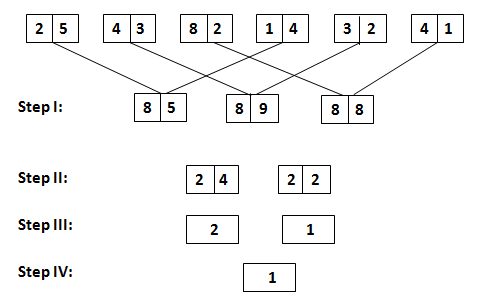# Input-Output Practice Set Based on SBI PO MainsThese questions are based on new pattern of reasoning question which is asked in SBI PO Mains 2017. This is similar to machine input. 3 questions were asked from this topic and each question contained 2 marks.

Directions (Q.1-3): The first step has been obtained by multiplying the digits in input. No other step uses multiplication concept. They are obtained by applying certain logic. Numbers of step II have been obtained by using at least 1 digit of each number in step I. Each step is a resultant of previous step.Q.1 What is the sum of second digit of largest number and first digit of smallest number in step I?
A. 15
B. 20
C. 17
D. 22
E. 25

Q.2 Which of the following will be step IV?
A. 1.5
B. 1
C. 2
D. 3
E. 4

Q.3 What is sum of numbers in step III?
A. 2
B. 2.5
C. 4
D. 3
E. 1.5

1. Ans. C.
Solution:
Largest Number=89, Smallest Number=85
9+8=17

2.Ans.
Solution:
Step IV: 1

3. Ans. D.
Solution:
2+1=3Step I: 2×4=8 5×1=5 , 4×2=8 3×3=9 , 8×1=8 2×4=8
Step II: 8+8+8=24 , 5+9+8=22
Step III: 4/2=2 , 2/2=1
Step IV: 2-1=1